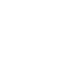# SAT Question of the Day (ACT too!): Sept. 22, 2014

If you are reading this in an email you received from me, do not click the link to sat.collegeboard.org below. Use the link to my website that is farther down on the email. If you are seeing this in my blog, do the SAT Question of the Day by clicking on this link:

http://sat.collegeboard.org/practice/sat-question-of-the-day?questionId=20130922&oq=1 (This link takes you to today’s question. If you use my archive, you will see the question related to my SAT explanation for that date.)

The answer is C.  The test writer’s explanation is unnecessarily complicated.  This is a simple slope question.  The slope of the line is rise/run or 8/12.  That reduces to 2/3 for y/x.  Remember that slope is a simple ratio expressed as a fraction.   This ratio is 2:3;  y goes up 2 units for every 3 units x increases.  Each time you go the right 3 units, you go up 2 units.

I think of it as a series of right triangles with a base of 3 and a height of 2.   The original line creates the hypotenuse.  For example, if you start at 0,0 and have a right triangle of base=3 and height=2, you are at coordinate 3,2.  Now put another right triangle starting right there.   You end up at 6,4 and so on.  What answer has a y/x value of 2/3?  Answer C.

Yes, you can use their approach of doing the algebra: y=2/3 of x.  But why?  It is a simple proportion question.  8/12=y/x=2/3.  Answer C.  All done.

What can you learn about the test?  The test writers like coordinate geometry.  Some of their questions will be simple slope questions like this one.  Video #8 also explains the other math they like to ask about coordinate geometry, for example, distance.  Sometimes they turn the questions into directions, like somebody drove north and then went west.  How do you easily find a bunch of actual sample coordinate geometry/grid questions?  First, get the Official SAT Study Guide.  Then look at the homework assignments on Demystifying the SAT & ACT.  I have analyzed all the questions in six tests and classified them by math concept.  Look at the ends of each lesson and at the end of the chapters.  You’ll easily find appropriate practice questions for the math topics that show up on the test.  Take advantage of my program and you’ll quickly customize your test prep and improve your score.

Let’s see what the ACT folks have for us today.

The answer is J.  Read the test writer’s explanation because they do a good job of explaining the composition rule.  It also saves me some typing!  So, let’s use the question to discuss how to approach the SAT English Test.  Disregard the “directions” that tell you to read the whole passage before beginning to answer questions.  Hogwash!  That would be an enormous waste of time.  Simply start reading the passage and do the questions as you come to them.  Let’s use this question, for example, to make my point!  You can read the sentence containing #12 in isolation of the rest of the passage and still get the question correct.  To be fair, for a few questions you may have to read a few sentences to get a context for the underlined word or words.  But to read the whole passage first is just terrible advice.

The SAT & ACT Wizard